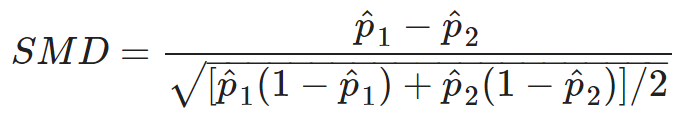OHDSI Home | Forums | Wiki | Github# How is the standard difference calculated in Atlas Characterization?

Hello.

I’m watching a tutorial on Atlas… and they say the standard difference is calculated from the percentages.
How is it calculated?
Their difference divided by the standard deviation of what?

I’ve found the equation.

It’s this one but without the division by two.t